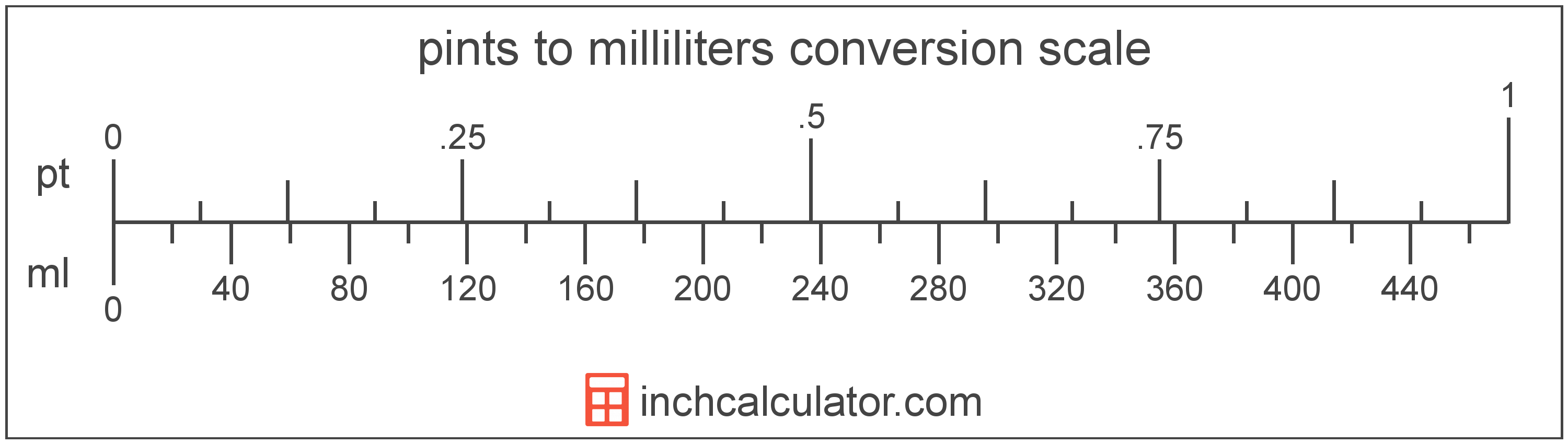# Pints to Milliliters Conversion

Enter the volume in pints below to get the value converted to milliliters.

Results in Milliliters:1 pt = 473.176473 ml

## How to Convert Pints to MillilitersTo convert a pint measurement to a milliliter measurement, multiply the volume by the conversion ratio.

Since one pint is equal to 473.176473 milliliters, you can use this simple formula to convert:

milliliters = pints × 473.176473

The volume in milliliters is equal to the pints multiplied by 473.176473.

For example, here's how to convert 5 pints to milliliters using the formula above.
5 pt = (5 × 473.176473) = 2,365.882365 ml

## Pints

The US liquid pint is a unit of fluid volume equal to one-eighth of a gallon, one-half of a quart, or two cups. The liquid pint should not be confused with the dry pint (US) or the imperial pint, which are different units.

The pint is a US customary unit of volume. Pints can be abbreviated as pt; for example, 1 pint can be written as 1 pt.

## Milliliters

The milliliter is a unit of volume equal to 1 cubic centimeter, 1/1,000 of a liter, or about 0.061 cubic inches.

The milliliter is an SI unit of volume in the metric system. In the metric system, "milli" is the prefix for 10-3. A milliliter is sometimes also referred to as a millilitre. Milliliters can be abbreviated as ml, and are also sometimes abbreviated as mL or mℓ. For example, 1 milliliter can be written as 1 ml, 1 mL, or 1 mℓ.

Milliliters are sometimes expressed using the abbreviation cc for medical dosage, which is the abbreviation for the cubic centimeter.

## Pint to Milliliter Conversion Table

Pint measurements converted to milliliters
Pints Milliliters
1 pt 473.18 ml
2 pt 946.35 ml
3 pt 1,420 ml
4 pt 1,893 ml
5 pt 2,366 ml
6 pt 2,839 ml
7 pt 3,312 ml
8 pt 3,785 ml
9 pt 4,259 ml
10 pt 4,732 ml
11 pt 5,205 ml
12 pt 5,678 ml
13 pt 6,151 ml
14 pt 6,624 ml
15 pt 7,098 ml
16 pt 7,571 ml
17 pt 8,044 ml
18 pt 8,517 ml
19 pt 8,990 ml
20 pt 9,464 ml
21 pt 9,937 ml
22 pt 10,410 ml
23 pt 10,883 ml
24 pt 11,356 ml
25 pt 11,829 ml
26 pt 12,303 ml
27 pt 12,776 ml
28 pt 13,249 ml
29 pt 13,722 ml
30 pt 14,195 ml
31 pt 14,668 ml
32 pt 15,142 ml
33 pt 15,615 ml
34 pt 16,088 ml
35 pt 16,561 ml
36 pt 17,034 ml
37 pt 17,508 ml
38 pt 17,981 ml
39 pt 18,454 ml
40 pt 18,927 ml

## References

1. National Institute of Standards and Technology, Specifications, Tolerances, and Other Technical Requirements for Weighing and Measuring Devices, Handbook 44 - 2019 Edition, https://nvlpubs.nist.gov/nistpubs/hb/2019/NIST.HB.44-2019.pdf